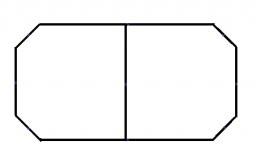# Right-angled 66364

From a rectangular board with dimensions of 2 m and 3 m, we cut isosceles right-angled triangles at the corners with an overhang of 40 cm. Calculate the ratio of the areas of the rest of the board to its total original area.

x =  71:75

### Step-by-step explanation:Did you find an error or inaccuracy? Feel free to write us. Thank you!

Tips for related online calculators
Need help calculating sum, simplifying, or multiplying fractions? Try our fraction calculator.
Check out our ratio calculator.
Calculation of an isosceles triangle.